# Polar coordinates

The numbersand(see ) related to rectangular Cartesian coordinatesandby the formulas: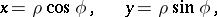where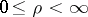,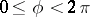. The coordinate lines are: concentric circles () and rays (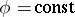).Figure: p073410a

The system of polar coordinates is an orthogonal system. To each point in the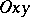-plane (except the pointfor whichandis undefined, i.e. can be any number) corresponds a pair of numbers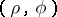and vice versa. The distancebetween a pointand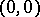(the pole) is called the polar radius, and the angleis called the polar angle. The Lamé coefficients (scale factors) are: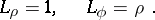The surface element is:The fundamental operations of vector analysis are: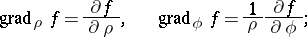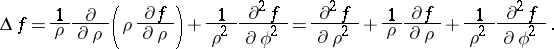The numbersandrelated to Cartesian rectangular coordinatesandby the formulas: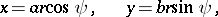where,,,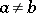, are called generalized polar coordinates. The coordinate lines are: ellipses (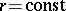) and rays ().